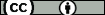## Boundary layers in a two-point boundary value problem with a caputo fractional derivative

Stynes, M. ; Gracia, J.L. (Universidad de Zaragoza)
Resumen: A two-point boundary value problem is considered on the interval [0, 1], where the leading term in the differential operator is a Caputo fractional derivative of order ¿ with 1 < ¿ < 2. Writing ¿ for the solution of the problem, it is known that typically ¿¿¿(¿) blows up as ¿ ¿ 0. A numerical example demonstrates the possibility of a further phenomenon that imposes difficulties on numerical methods: ¿ may exhibit a boundary layer at ¿ = 1 when ¿ is near 1. The conditions on the data of the problem under which this layer appears are investigated by first solving the constant-coefficient case using Laplace transforms, determining precisely when a layer is present in this special case, then using this information to enlighten our examination of the general variable-coefficient case (in particular, in the construction of a barrier function for ¿). This analysis proves that usually no boundary layer can occur in the solution ¿ at ¿ = 0, and that the quantity ¿ = max¿¿[0,1] ¿(¿), where ¿ is the coefficient of the first-order term in the differential operator, is critical: when¿ < 1,noboundarylayerispresentwhen¿isnear1,butwhen¿ = 1thenaboundarylayerat¿ = 1 is possible. Numerical results illustrate the sharpness of most of our results.
Idioma: Inglés
DOI: 10.1515/cmam-2014-0024
Año: 2015
Publicado en: Computational Methods in Applied Mathematics 15, 1 (2015), 79-95
ISSN: 1609-4840

Factor impacto JCR: 0.673 (2015)
Categ. JCR: MATHEMATICS, APPLIED rank: 163 / 254 = 0.642 (2015) - Q3 - T2
Factor impacto SCIMAGO: 0.901 - Applied Mathematics (Q2) - Numerical Analysis (Q2) - Computational Mathematics (Q2)

Financiación: info:eu-repo/grantAgreement/ES/MEC/MTM2010-16917
Tipo y forma: Article (Published version)You must give appropriate credit, provide a link to the license, and indicate if changes were made. You may do so in any reasonable manner, but not in any way that suggests the licensor endorses you or your use.

Este artículo se encuentra en las siguientes colecciones:
Articles